# 随版本更新的装备推荐（12.02更新所有核心数据装备）

## 前言及引用资料

8.12更新：感谢群内大佬更新射速计算公式，游戏真正的射速计算公式为：

8.14更新：感谢群内@无远弗届 的测试，阵型的炮击值和雷击值buff不会给后排舰队，但轮形阵的防空buff有效。

8.16更新：新增战列炮命中系数，重新修改BBBC部分。引用自[战术学院] 命中与BB的不寻常关系·二的数据进行计算。

8.23更新：你游更新了一众数据。。。还有一票机制被挖出来。。。我觉得整篇文章都要改了。。。DPS计算部分计入主炮开火时间，此部分不受装填值影响。 计算量又大了好多 鱼雷效率更新，Z46加强了。。。德意志伤害计算等等。。。之前一直有人跟我说127体感不好，终于是找到原因了。。。我之前因为没有数据，只能按现有数据评测，所以没办法修改推荐，现在 整篇文章都废了

9.29更新：秋活又是一波大改，更新一众数据，更新计算DPS的方法，现在所有舰炮对轻中重甲的补正计算比例，轻甲系数*1.2，中甲*1.1，重甲*0.7，然后相加除以3

10.3：编辑到一半，一不小心关网页了，改动的数据都没了。。。。不活了

12.2更新：终于是把之前差的装备补上了。然而还有很多得改的没改。。。算了以后再说吧

12.30更新：装备有高论（顺口溜的方式，萌新不知装啥好，来看装备有高论！）

## 主炮篇

### DD主炮

DD主炮主要有四门，分别是

：(7*1.15*(1+82/100)*1*(1*1.2+0.5*1.1+0.2*0.7)/3*1.25)/(0.08+0.1+0+(0.67*25.06/(3.14*201+314)^0.5))=15.89，弹幕DPS为：(12*12*3*(1+82/100)*1*1.2*1)/((0.08+0.1+0+(0.67*25.06/(3.14*201+314)^0.5))*10)=129.93，命中率为125%时，DPS为162.41，总DPS为178.30，命中率为85%时，DPS为110.44，总DPS为126.33

：(12*4*1.15*(1+85/100)*1*(1*1.2+0.5*1.1+0.2*0.7)/3*1.1)/(0.16+0.1+0.1+(0.96*25.06/(3.14*201+314)^0.5))=61.94，弹幕DPS为：(12*12*3*(1+85/100)*1*1.2*1)/((0.16+0.1+0.1+(0.96*25.06/(3.14*201+314)^0.5))*10)=83.40，命中率为125%时，DPS为104.92，总DPS为166.87，命中率为85%时，DPS为71.35，总DPS为133.29

：(15*4*1.15*(1+90/100)*1*(1.2*1.2+0.6*1.1+0.6*0.7)/3*1.1)/(0.16+0.1+0.1+(1.53*25.06/(3.14*201+314)^0.5))=75.37，弹幕DPS为：(12*12*3*(1+90/100)*1*1.2*1)/((0.16+0.1+0.1+(1.53*25.06/(3.14*201+314)^0.5))*10)=61.29，命中率为125%时，DPS为76.61，总DPS为151.98，命中率为85%时，DPS为52.09，总DPS为127.46

：(15*3*1.15*(1+95/100)*1*(1.2*1.2+0.6*1.1+0.6*0.7)/3*1.25)*0.7/(0.16+0.1+0.3+(1.06*25.06/(3.14*201+314)^0.5))=52.08，弹幕DPS为：(12*12*3*(1+95/100)*1*1.2*1)/((0.16+0.1+0.3+(1.06*25.06/(3.14*201+314)^0.5))*10)=70.99，命中率为125%时，DPS为88.73，总DPS为140.82，命中率为85%时，DPS为60.34，总DPS为112.42

：(15*4*1.15*(1+95/100)*1*(1*1.2+0.5*1.1+0.2*0.7)/3*1.25)/(0.16+0.1+0.1+(1.44*25.06/(3.14*201+314)^0.5))=69.08，弹幕DPS为：(12*12*3*(1+109/100)*1*1.2*1)/((0.16+0.1+0.1+(1.44*25.06/(3.14*201+314)^0.5))*10)=65.91，命中率为125%时，DPS为82.38，总DPS为151.47，命中率为85%时，DPS为56.02，总DPS为125.10

：(7*1.6*(1+90/100)*1*(1*1.2+0.5*1.1+0.2*0.7)/3*1.25)/(0.08+0.1+0+(0.67*25.06/(3.14*184+314)^0.5))=22.58，弹幕DPS为：(12*48*(1+90/100)*1*(0.9*1.2+0.7*1.1+0.4*0.7)/3*1.25)*0.75/((0.08+0.1+0+(0.67*25.06/(3.14*184+314)^0.5))*10)=98.14，一级技能计算命中DPS为112.86，总DPS为135.44，满级技能计算命中DPS为122.68，总DPS为145.25

：(15*3*1.6*(1+103/100)*1*(1.2*1.2+0.6*1.1+0.6*0.7)/3*1.25)*0.7/(0.16+0.1+0.3+(1.06*25.06/(3.14*184+314)^0.5))=74.11，弹幕DPS为(12*48*(1+103/100)*1*(0.9*1.2+0.7*1.1+0.4*0.7)/3*1.25)*0.75/((0.16+0.1+0.3+(1.06*25.06/(3.14*184+314)^0.5))*10)=53.69，一级技能计算命中DPS为61.75，总DPS为135.86，满级技能计算命中DPS为67.12，总DPS为141.23

：12*4*1.6*(1+93/100)*1*(1*1.2+0.5*1.1+0.2*0.7)/3*1.1)/(0.16+0.1+0.1+(0.96*25.06/(3.14*184+314)^0.5))=88.12，弹幕DPS为(12*48*(1+93/100)*1*(0.9*1.2+0.7*1.1+0.4*0.7)/3*1.25)*0.75/((0.16+0.1+0.1+(0.96*25.06/(3.14*184+314)^0.5))*10)=63.48，一级技能计算命中DPS为73.00，总DPS为161.13，满级技能计算命中DPS为79.35，总DPS为167.48

：(15*4*1.6*(1+98/100)*1*(1.2*1.2+0.6*1.1+0.6*0.7)/3*1.1)/(0.16+0.1+0.1+(1.53*25.06/(3.14*184+314)^0.5))=106.84，弹幕DPS为(12*48*(1+98/100)*1*(0.9*1.2+0.7*1.1+0.4*0.7)/3*1.25)*0.75/((0.16+0.1+0.1+(1.53*25.06/(3.14*184+314)^0.5))*10)=46.18，一级技能计算命中DPS为53.10，总DPS为159.94，满级技能计算命中DPS为57.72，总DPS为164.56

：(11*4*1.6*(1.93/100)*1*(0.9*1.2+0.7*1.1+0.4*0.7)/3*1.15)*1.25/(0.16+0.1+0.1+(1.50*25.06/(3.14*184+314)^0.5))=87.89，弹幕DPS为(12*48*(1+93/100)*1*(0.9*1.2+0.7*1.1+0.4*0.7)/3*1.25)*0.75/((0.16+0.1+0.1+(1.53*25.06/(3.14*184+314)^0.5))*10)=45.71，一级技能计算命中总DPS为153.64，满级技能计算命中总DPS为167.00

：(15*4*1.6*(1+103/100)*1*(1*1.2+0.5*1.1+0.2*0.7)/3*1.25)/(0.16+0.1+0.1+(1.44*25.06/(3.14*184+314)^0.5))=97.85，弹幕DPS为(12*48*(1+103/100)*1*(0.9*1.2+0.7*1.1+0.4*0.7)/3*1.25)*0.75/((0.16+0.1+0.1+(1.44*25.06/(3.14*184+314)^0.5))*10)=47.18，一级技能计算命中DPS为54.26，总DPS为152.10，满级技能计算命中DPS为58.97，总DPS为156.82

DPS：(156*5*1.5*(1+540/100)*(0.8+1.0+1.3)/3)/(26.44*(200/(197+100))^0.5)=356.62，计算命中率DPS为213.97，加上炮击输出总DPS为306.61

DPS：(156*5*1.3*(1+386/100)*(0.8+1.0+1.3)/3)/(26.44*(200/(201+100))^0.5)=236.28，计算命中率DPS为141.77，加上最高炮击输出总DPS为320.07

DPS：(156*5*1.2*(1+455/100)*(0.8+1.0+1.3)/3)/(26.44*(200/(184+100))^0.5)=241.93，计算命中率DPS为145.16，加上最高炮击输出总DPS为312.64

i的DPS在未被技能buff的情况下达到了306左右，若计算技能buff(实际增伤可达30%左右)，DPS可达到恐怖的400左右。

Z23你根本不是DD！展开/折叠

，此处假设命中率为70%：(15*3*1.6*(1+143*(1+0.1+0.2+0.1752)/100)*1*(1.2*1.2+0.6*1.1+0.6*0.7)/3*1.25)*0.7/1.3694=120.17，弹幕DPS为(12*18*(1+143*(1+0.1+0.2+0.1752)/100)*1*(1*1.2+0.8*1.1+0.6*0.7)/3*1.25)*0.4/13.694=20.44，总DPS为140.61

(15*4*1.6*(1+143*(1+0.1+0.2+0.1644)/100)*1*(1*1.2+0.5*1.1+0.2*0.7)/3*1.25)/1.4596=160.26，弹幕DPS为(12*18*(1+143*(1+0.1+0.2+0.1644)/100)*1*(1*1.2+0.8*1.1+0.6*0.7)/3*1.25)*0.4/14.596=19.08，总DPS为179.34

(15*4*1.6*(1+1.38*(1+0.1+0.2+0.1570)/100)*1*(1.2*1.2+0.6*1.1+0.6*0.7)/3*1.1)/1.5283=174.74，弹幕DPS为(12*18*(1+138*(1+0.1+0.2+0.1570)/100)*1*(1*1.2+0.8*1.1+0.6*0.7)/3*1.25)*0.4/15.283=17.73，总DPS为192.47

，此处假设命中率为125%：(21*4*1.6*(1+143*(1+0.1+0.2+0.0905)/100)*1*(1*1.2+0.8*1.1+0.6*0.7)/3*1.05)*1.25/2.6508=165.72，弹幕DPS为(12*18*(1+143*(1+0.1+0.2+0.0905)/100)*1*(1*1.2+0.8*1.1+0.6*0.7)/3*1.25)*0.4/26.508=10.15，总DPS为175.87

### CL主炮

PS：我用满级海妈分别装备+7贝爷炮和+6克爹炮(其他装备相同)分别打了3-4boss，结果两次时间均为59s。

### CA主炮

：50*6*1.4*(1+324*1.15/100)*(0.75*1.2+1.1*1.1+0.75*0.7)/3*1.1/(7.65*25.06/(3.14*223+314)^0.5+0.2+1.0+0.1)=262.00，弹幕总伤害为40*12*(1+324*1.15/100)*(0.75*1.2+1.1*1.1+0.75*0.7)/3*1*0.75+40*11*(1+324*1.15/100)*(1*1.2+0.8*1.1+0.6*0.7)/3*1*0.7=2707.37，每43.92s发射一次。

：55*6*1.4*(1+304*1.15/100)*(0.75*1.2+1.1*1.1+0.75*0.7)/3*1.1/(8.98*25.06/(3.14*223+314)^0.5+0.2+0.5+0.1)=255.12，弹幕总伤害为40*12*(1+304*1.15/100)*(0.75*1.2+1.1*1.1+0.75*0.7)/3*1*0.75+40*11*(1+304*1.15/100)*(1*1.2+0.8*1.1+0.6*0.7)/3*1*0.7=2575.61，每47.20s发射一次。

：52*3*1.4*(1+304*1.15/100)*(1.35*1.2+0.95*1.1+0.7*0.7)/3*1.05*0.7/(5.59*25.06/(3.14*223+314)^0.5+0.2+0+0.1)=161.53，弹幕总伤害为40*12*(1+304*1.15/100)*(0.75*1.2+1.1*1.1+0.75*0.7)/3*1*0.75+40*11*(1+304*1.15/100)*(1*1.2+0.8*1.1+0.6*0.7)/3*1*0.7=2575.61，每28.19s发射一次。

：48*4*1.4*(1+304*1.15/100)*(1.35*1.2+0.95*1.1+0.7*0.7)/3*1.1/(5.92*25.06/(3.14*223+314)^0.5+0.2+0.5+0.1)=256.13，弹幕总伤害为40*12*(1+324*1.15/100)*(0.75*1.2+1.1*1.1+0.75*0.7)/3*1*0.75+40*11*(1+324*1.15/100)*(1*1.2+0.8*1.1+0.6*0.7)/3*1*0.7=2707.37，每32.75s发射一次

203试作是203连装的上位替代，两炮的差距虽然不大，但也不小。可以说是目前最适合德意志的主炮。

### BB主炮

BB主炮机制复杂，如果你不想看分析，可以无脑选择MK6(我就是这么干的)，在这里对5门主炮作出分析。

8.16更新：引入期望伤害计算，具体请看命中与BB的不寻常关系

，DPS为 28.644/22.506/18.414，计算期望补正后DPS为DPS*0.487=13.950/10.960/8.968

，DPS为 23.688/18.612/15.288 ，计算期望补正后DPS为DPS*0.572=13.550/10.646/8.755

，DPS为 4.737/20.527/17.369，计算期望补正后DPS为DPS*0.572=2.710/11.741/9.935

，DPS为 11.669/16.67/15.003 ，计算期望补正后DPS为DPS*0.49=5.664/8.168/7.351

，DPS为 22.806/17.919/14.661 ，因为这门炮散布为22，故子弹命中率按三联算，计算期望补正后DPS为DPS*0.49=11.175/8.780/7.184

410是作为MK6的备选主炮出现，410由于舰炮补正优势，点燃伤害比MK6高，加上射速不慢，可以说是稳坐BB第二高爆炮的位置，是MK6的下位替代(PVP中可以取代MK6)

380则是381的升级版，在PVP中通常比MK5好用。MK5是单轮伤害最高的穿甲主炮。

381开一轮炮的总伤(计算0.41演习场22散布BB炮命中期望)为2081，对中甲补正后为2289，对重甲补正后为1873，点燃伤害为639/3s，点燃总伤为3195

## 舰载机篇

### 战斗机（舰战）篇

8.22版本更新后，海盗被增强为携带两枚500lb航弹，可以说是一跃成为第一紫色战斗机

### 轰炸机（舰爆）

100lb范围(半径)为1

500lb范围(半径)为1.1875

1000lb范围(半径)为1.375

1600lb范围(半径)为1.5625

2000lb范围(半径)为1.75

100lb范围(半径)为1

500lb范围(半径)为1.091

1000lb范围(半径)为1.212

1600lb范围(半径)为1.212

2000lb范围(半径)为1.273

### 鱼雷机（舰攻）

PS：紫色流星，除了射速，也全面优于天山，在不争分夺秒的情况下，优先紫色流星。（前提是你要有）

TBF由于其高鱼雷伤害和低放飞速度，在对CD时间要求不高且没有金梭鱼用的时候，可以考虑使用TBF。

## 设备篇

1.

 SG雷达T3 适用舰种 驱逐、轻巡、重巡、超巡、战巡、战列、重炮、维修、潜艇、航战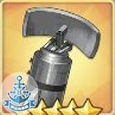命中 初始 14 机动 初始 6 强满 35 强满 15 技能 提供了极其优秀的侦查，受到伏击概率降低12%，规避伏击概率提高12%，效果不可叠加。 所属科技箱 克洛希德 设计图掉落 6-1、10-2、坠落之翼C2、光与影的鸢尾之华D1、复刻坠落之翼C2、复刻光与影的鸢尾之华D1、箱庭疗法C3、神圣的悲喜剧C1

SG雷达可以说是你游神装之一，光是伏击概率降低和规避概率提高这个技能，就奠定了SG的地位，反而是属性倒是变成了锦上添花的东西。命中属性其实无关紧要，倒是机动属性在PVE中大放光彩。

2.

 九三式纯氧鱼雷T3 适用舰种 驱逐、轻巡、重巡、超巡、潜艇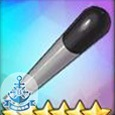雷击 初始 40 装填 初始 3 强满 100 强满 10 技能 所属科技箱 核心兑换 设计图掉落

3.

 对空雷达T3 适用舰种 驱逐、轻巡、重巡、超巡、战巡、战列、航母、轻航、重炮、维修、潜艇、航战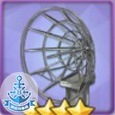防空 初始 28 强满 70 技能 所属科技箱 通用 设计图掉落 2-3、2-4、异色格B2、异色格D2、坠落之翼A2、坠落之翼C2、复刻异色格B2、复刻异色格D2、神圣的悲喜剧A1、神圣的悲喜剧C1、穹顶下的圣咏曲C1、蝶海梦花T5、蝶海梦花HT5

4.

 小海狸中队队徽T1 适用舰种 驱逐、轻巡、重巡、超巡、战巡、战列、航母、轻航、重炮、维修、潜艇、航战机动 初始 14 耐久 初始 25 强满 35 强满 75 技能 小海狸中队：全队航速提高20% 所属科技箱 无 设计图掉落 收藏：查尔斯·奥斯本、撒切尔、奥利克、富特、斯彭斯（合计满17星解锁）

20%航速提升是什么概念呢？我们假设三船前锋，双CA一CL，航速大约是(25+25+33)/3=27.67，大约没比一只CA快多少，但是带了小海狸之后，27.67*1.2=33.2，确确实实达到了轻巡水准，可以说是非常好用。

5.

 改良锅炉T3 适用舰种 驱逐、轻巡、重巡、超巡、战巡、战列、航母、轻航、重炮、维修、潜艇、航战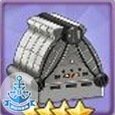耐久 初始 98 航速 初始 3 强满 245 强满 8 技能 所属科技箱 通用 设计图掉落 5-1、9-1、红染A2、红染C2、坠落之翼C1、围剿SP1、异次元SP3、凛冬王冠B3、光与影的鸢尾之华A3、光与影的鸢尾之华C3、传颂之物SP4、苍红的回响B2、苍红的回响D2、复刻光与影的鸢尾之华A3、复刻光与影的鸢尾之华C3、箱庭疗法B3、箱庭疗法D3、箱庭疗法SP、神圣的悲喜剧B1、神圣的悲喜剧D1、虚拟与真实的双向镜T4、浮樱影华B2、浮樱影华D2、北境序曲C1、复刻苍红的回响B2、复刻苍红的回响D2、穹顶下的圣咏曲B1、穹顶下的圣咏曲D1、峡湾间的反击SP3

6.

 治愈系猫爪T1 适用舰种 维修、驱逐、轻巡、重巡、超巡、战巡、战列、航母、轻航、重炮、潜艇、航战耐久 初始 120 强满 300 技能 喵喵治愈：明石装备时生效)所有治疗效果提高20%，不可叠加 所属科技箱 设计图掉落

 液压舵机T3 适用舰种 驱逐、轻巡、重巡、超巡、战巡、战列、航母、轻航、重炮、维修、潜艇、航战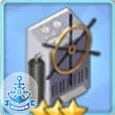机动 初始 9 耐久 初始 20 强满 17 强满 44 技能 所属科技箱 通用 设计图掉落 2-1、异次元SP1、铁血音符誓言A2、穿越彼方的水线、复刻特别演习·埃塞克斯级、复刻铁血音符誓言A2

8.

 液压弹射装置T3 适用舰种 航母、轻航、航战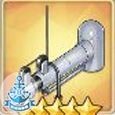航空 初始 40 耐久 初始 25 强满 100 强满 75 技能 所属科技箱 通用 设计图掉落 坠落之翼D3、墨染D2、复刻坠落之翼D3、复刻墨染D2、北境序曲D2

T2版本的紫装也不错，强满70航空值，也不少，是没有金液压和紫油箱时的选择。

9.

 火控雷达T3 适用舰种 重巡、超巡、战巡、战列、重炮、航战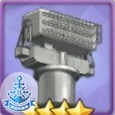命中 初始 9 炮击 初始 8 强满 24 强满 21 技能 所属科技箱 通用 设计图掉落 4-3、10-3、铅色SP2、坠落之翼B1、坠落之翼D1、凛冬王冠A3、凛冬王冠C3、墨染B2、墨染D2、传颂之物SP4、苍红的回响A3、苍红的回响C3、复刻墨染B2、复刻墨染D2、铁血音符誓言B3、箱庭疗法B1、箱庭疗法D1、神圣的悲喜剧B3、神圣的悲喜剧D3、神圣的悲喜剧SP、浮樱影华B3、浮樱影华D3、北境序曲B3、北境序曲D3、微层混合B1、微层混合D1、微层混合SP、复刻苍红的回响A3、复刻苍红的回响C3、穹顶下的圣咏曲B3、穹顶下的圣咏曲D3、穹顶下的圣咏曲SP、永夜幻光D3、复刻铁血音符誓言B3、蝶海梦花T6、蝶海梦花HT6、蝶海梦花SP

10.

 灭火器T3 适用舰种 驱逐、轻巡、重巡、超巡、战巡、战列、航母、轻航、重炮、维修、潜艇、航战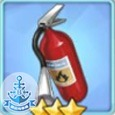耐久 初始 140 强满 266 技能 起火概率降低30%，持续时间缩短6秒，起火伤害降低20%，同类效果取最大值，不可叠加 所属科技箱 通用 设计图掉落 2-3、光与影的鸢尾之华A3、复刻光与影的鸢尾之华A3、浮樱影华A2、永夜幻光A2、蝶海梦花T1

11.

 燃油滤清器T3 适用舰种 驱逐、轻巡、重巡、超巡、战巡、战列、航母、轻航、重炮、维修、潜艇、航战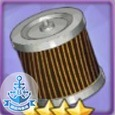耐久 初始 140 机动 初始 2 强满 350 强满 5 技能 所属科技箱 通用 设计图掉落 3-1、9-2、10-4、坠落之翼A3、坠落之翼C3、围剿SP2、凛冬王冠A2、凛冬王冠C2、墨染C1、苍红的回响A3、苍红的回响C3、复刻墨染C1、虚拟链接的共时性SP3、浮樱影华C2、复刻苍红的回响A3、复刻苍红的回响C3、蝶海梦花HT2

12.

 珍珠之泪T1 适用舰种 驱逐、轻巡、重巡、超巡、战巡、战列、航母、轻航、重炮、维修、潜艇、航战耐久 初始 200 强满 500 技能 珍珠之泪：被击沉时恢复全队其他角色耐久10% 所属科技箱 无 设计图掉落 收藏：亚利桑那、俄克拉荷马、卡辛、唐斯（合计满13星解锁）

13.

 电动扬弹机T3 适用舰种 驱逐、轻巡、重巡、超巡、战巡、战列、重炮、维修、潜艇、航战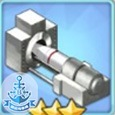装填 初始 9 炮击 初始 3 强满 17 强满 5 技能 所属科技箱 通用 设计图掉落 1-4、墨染B2、复刻墨染B2、箱庭疗法A1、峡湾间的反击SP1

14.

 维修工具T3 适用舰种 驱逐、轻巡、重巡、超巡、战巡、战列、航母、轻航、重炮、维修、潜艇、航战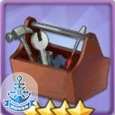耐久 初始 200 强满 500 技能 每15秒1.0%缓慢回复耐久，同类效果取最大值，不可叠加 所属科技箱 通用 设计图掉落 3-4、10-3、铅色SP3、坠落之翼B2、月光下的序曲SP3、苍红的回响B1、苍红的回响D1、铁血音符誓言B3、铁血音符誓言D3、铁血音符誓言SP、浮樱影华B2、浮樱影华D2、北境序曲C1、微层混合C2、复刻苍红的回响B1、复刻苍红的回响D1、穹顶下的圣咏曲B3、永夜幻光C2、复刻铁血音符誓言B3、复刻铁血音符誓言D3、复刻铁血音符誓言SP

15.

 航空副油箱T3 适用舰种 航母、轻航、航战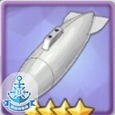航空 初始 28 耐久 初始 20 强满 70 强满 60 技能 舰载机耐久增加120点，同类效果取最大值，不可叠加 所属科技箱 通用 设计图掉落 5-3、异色格B2、异色格D2、凛冬王冠B2、复刻异色格B2、复刻异色格D2、铁血音符誓言A2、铁血音符誓言C2、神圣的悲喜剧A3、神圣的悲喜剧C3、虚拟与真实的双向镜T5、复刻特别演习·埃塞克斯级、复刻铁血音符誓言A2、复刻铁血音符誓言C2

16.

 舰艇维修设备T3 适用舰种 维修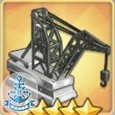耐久 初始 200 强满 500 技能 维修Lv3：每5秒回复15点耐久，同类效果取最大值，不可叠加(作用目标为1个，优先百分比血量最少的) 所属科技箱 通用 设计图掉落 9-2、红染C2、墨染C3、复刻墨染C3

17.

 链式装弹机T3 适用舰种 驱逐、轻巡、重巡、超巡、战巡、战列、重炮、维修、潜艇、航战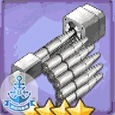装填 初始 14 炮击 初始 3 强满 35 强满 7 技能 所属科技箱 通用 设计图掉落 6-3、10-1、11-3、坠落之翼B1、坠落之翼D1、凛冬王冠B1、凛冬王冠D1、墨染C2、月光下的序曲SP2、苍红的回响B3、复刻墨染C2、箱庭疗法C1、神圣的悲喜剧B1、神圣的悲喜剧C1、神圣的悲喜剧D1、北境序曲C2、北境序曲SP、复刻苍红的回响B3、穹顶下的圣咏曲B2、穹顶下的圣咏曲D2

18.

 防鱼雷隔舱T3 适用舰种 轻巡、重巡、超巡、战巡、战列、航母、轻航、重炮、维修、航战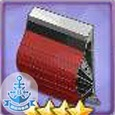耐久 初始 140 强满 350 技能 受到鱼雷伤害减少30%，同类效果取最大值，不可叠加 所属科技箱 通用 设计图掉落 4-1、7-4、10-2、墨染C3、月光下的序曲SP1、复刻墨染C3、铁血音符誓言B3、铁血音符誓言D3、铁血音符誓言SP、神圣的悲喜剧B3、神圣的悲喜剧D3、神圣的悲喜剧SP、虚拟与真实的双向镜T3、虚拟与真实的双向镜SP、浮樱影华A2、浮樱影华C2、北境序曲B3、穹顶下的圣咏曲B3、永夜幻光C2、复刻铁血音符誓言B3、复刻铁血音符誓言D3、复刻铁血音符誓言SP

19.

 陀螺仪T3 适用舰种 驱逐、轻巡、重巡、超巡、战巡、战列、重炮、维修、潜艇、航战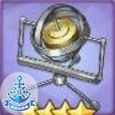命中 初始 14 航速 初始 3 强满 35 强满 8 技能 所属科技箱 通用 设计图掉落 6-2、10-2、七夕Sp3、坠落之翼A3、坠落之翼C3、凛冬王冠A2、凛冬王冠C2、神圣的悲喜剧B1、穹顶下的圣咏曲B1、峡湾间的反击SP3、永夜幻光D1、蝶海梦花T4、蝶海梦花HT4

20.

 “宁海号”水上侦察机T0 适用舰种 轻巡、重巡、超巡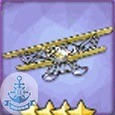命中 初始 15 强满 35 技能 空中侦查：放出宁海号进行侦查，受到伏击概率降低8%，规避伏击概率提高8%，效果不可叠加 所属科技箱 设计图掉落

21.

 九一式穿甲弹T0 适用舰种 战巡、战列、航战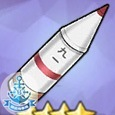炮击 初始 14 命中 初始 4 强满 35 强满 10 技能 九一式穿甲弹：主炮炮击的暴击伤害提升15% 所属科技箱 藏王重工 设计图掉落

22.

 一式穿甲弹T0 适用舰种 战巡、战列、航战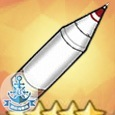炮击 初始 22 命中 初始 6 强满 55 强满 15 技能 一式穿甲弹：主炮炮击的暴击伤害提升25% 所属科技箱 核心兑换 设计图掉落

23.

 超重弹T0 适用舰种 战巡、战列、航战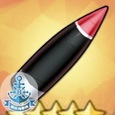炮击 初始 28 强满 70 技能 超重弹：主炮炮击的暴击率提高8% 所属科技箱 核心兑换 设计图掉落

## 常见舰娘装备初阶搭配

SG雷达可在除CV，CVL以外任意舰娘上装备，可让任意舰娘装备，当然，输出高的舰娘优先装备输出装。

DD篇展开/折叠

CL篇展开/折叠

CA篇展开/折叠

### BB篇

BB篇主炮参见上半部分及下面对格奈森瑙的分析。

BB篇展开/折叠

#### 格奈森瑙用什么主炮PVE输出更高？

380DPS为 40/176/149，每轮伤害为735/3236/2740

MK6依然是最泛用的主炮

## 后记

PS：感谢win10计算器对本文章的大力支持！

B站安卓服：朱诺行动，昵称：Mr777，所属大舰队：超凡